# Printable Number Chart 1 30Updated on Nov 11, 2022By Printablee Team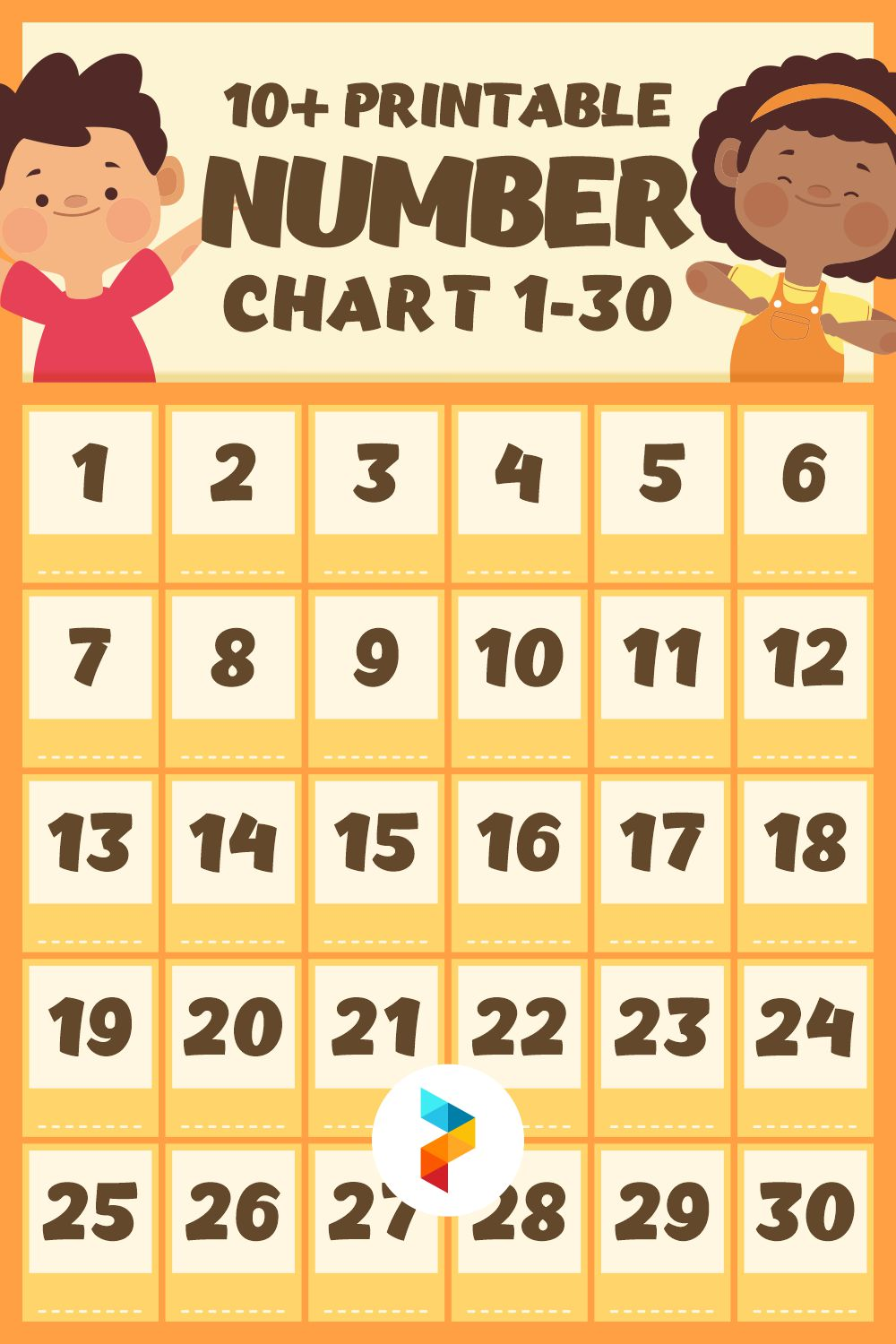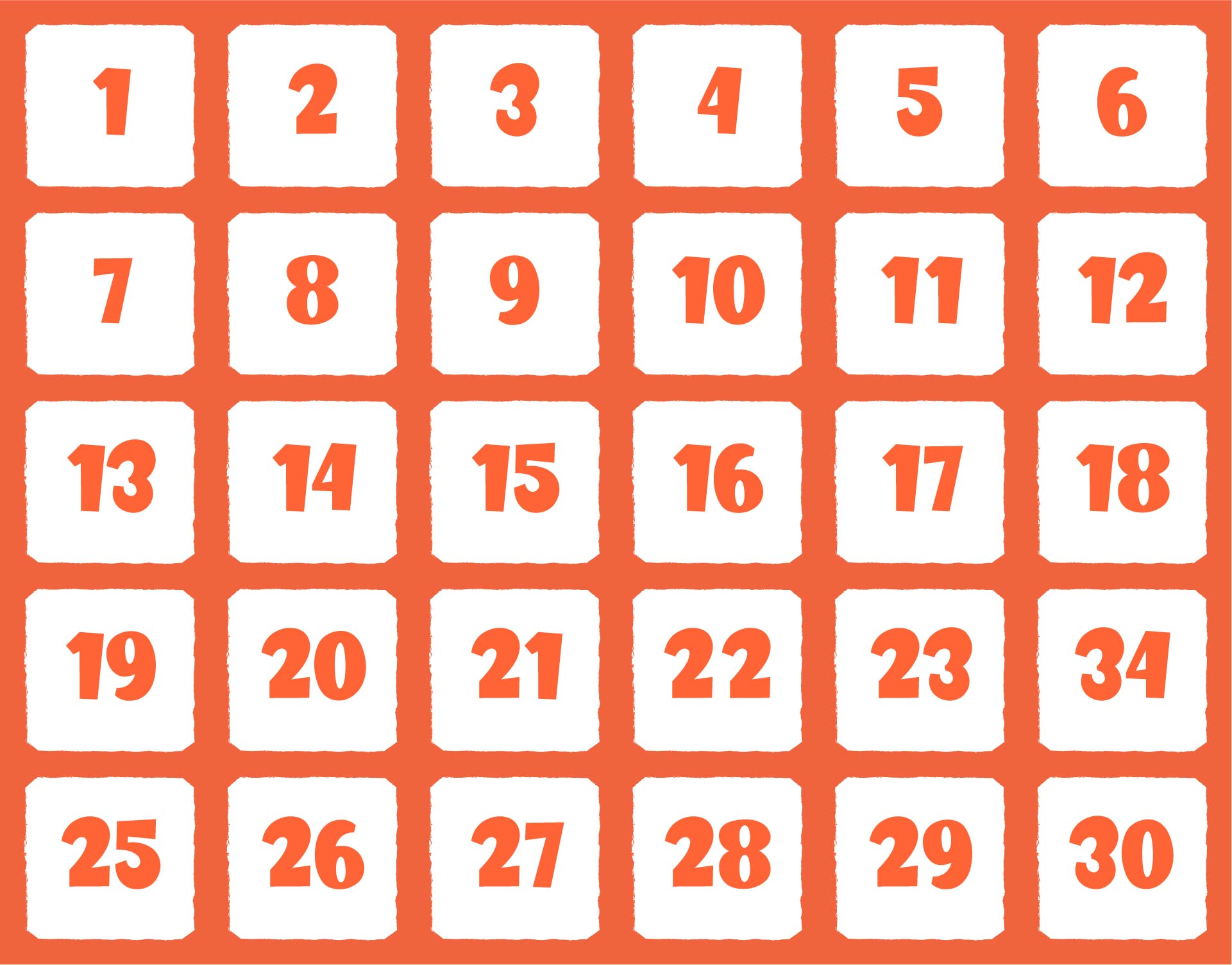## What is Meant by Mathematical Operations?

Mathematical operations are various ways that are used to calculate numbers. In basic mathematical operations, there are several calculations that are performed such as subtraction, addition, multiplication, and division.

• The addition is a combination or sum of two or more numbers that produce a new number.

• Subtraction is taking several numbers from a certain number so the numbers decrease.

• Multiplication is the addition of numbers repeatedly. Multiplication can be interpreted as the addition of the same number several times (according to how many multipliers there are).

• The division is subtraction done repeatedly. This is also interpreted by dividing the numbers into several groups of the same number.

Not only those arithmetic operations, mathematical operations also involve mixed arithmetic operations. So, the four types of arithmetic operations above are combined into one. If you find mixed arithmetic operations, then do these ways.

• If in the mixed arithmetic operation, there is subtraction and addition, what must be done first is the arithmetic operation on the left.

• If there is multiplication and division in the mixed arithmetic operations, do the arithmetic operations on the left first.

• If there are addition or subtraction operations that are combined with division or multiplication, what is done first is division or multiplication. Then, continue it by doing subtraction or addition.

• If there are numbers or arithmetic operations that are placed in parentheses, do the arithmetic operations in the parentheses first.

By understanding all of these rules, it will be easier for you to solve mathematical operation problems. This method can be used to teach basic mathematical operations to children.

## How to Teach Basic Mathematical Operations to Children?

You don't just need numbers to explain basic math operations to kids. These counting activities must be made as attractive as possible so children are interested in learning them and easy to understand these calculations. Therefore, you need to know how to guide kids to learn basic mathematic operations.

However, before doing some of these methods, you should introduce numbers to children. This will make it easier for children to do calculations. Once they have all the numbers memorized, move on to teaching basic math operations with these strategies.

• Objects around children: The first way to do this is to use objects that are around children. It is the easiest way to introduce various types of mathematical arithmetic operations. Teach children to count toys, fruit, vegetables, or other objects around the house. Teach subtraction, addition, multiplication, and division using these objects.

• Baking cakes: Another way is to use baked cookies. Ask the children to help you make the cookies. When printing those cookies, teach children basic math operations. Let them count the number of cookies. Then, put some of the cookies in the jar and ask the children to count the cookies that haven't been put in the jar.

• Math games: You can also use some types of math games with your kids. There are several games that use simple math concepts such as Monopoly and Yahtzee. You can also create your own games like asking the kids to run to the squares. Draw squares in chalk with the numbers 1-10. Then, give the children basic math operations problems. An example is 2+3 = 5. So, the children have to run to the box marked with the 5 number.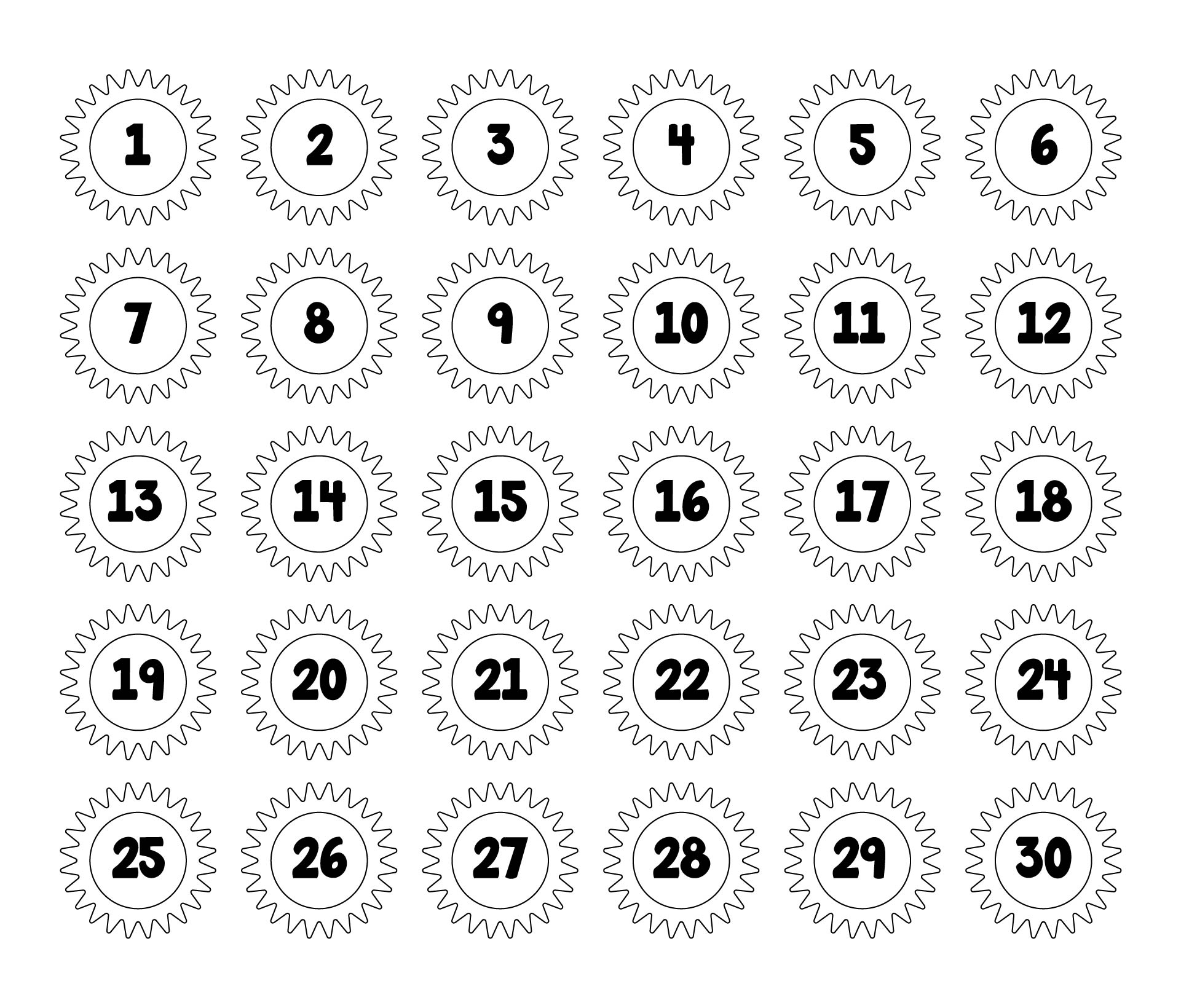We also have more printable chart you may like:
Printable Fraction Chart
Printable Sticker Charts
Printable House Cleaning Charts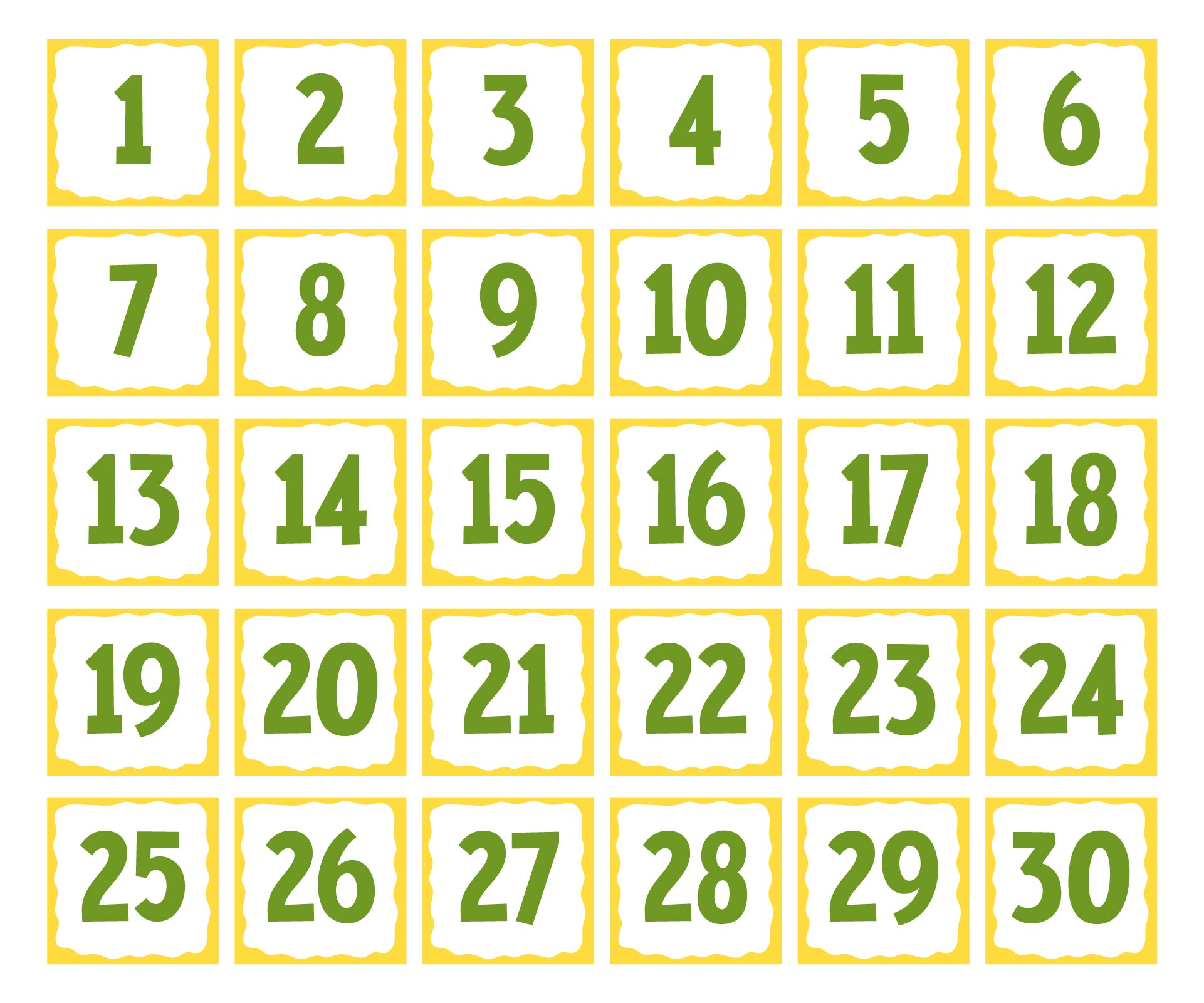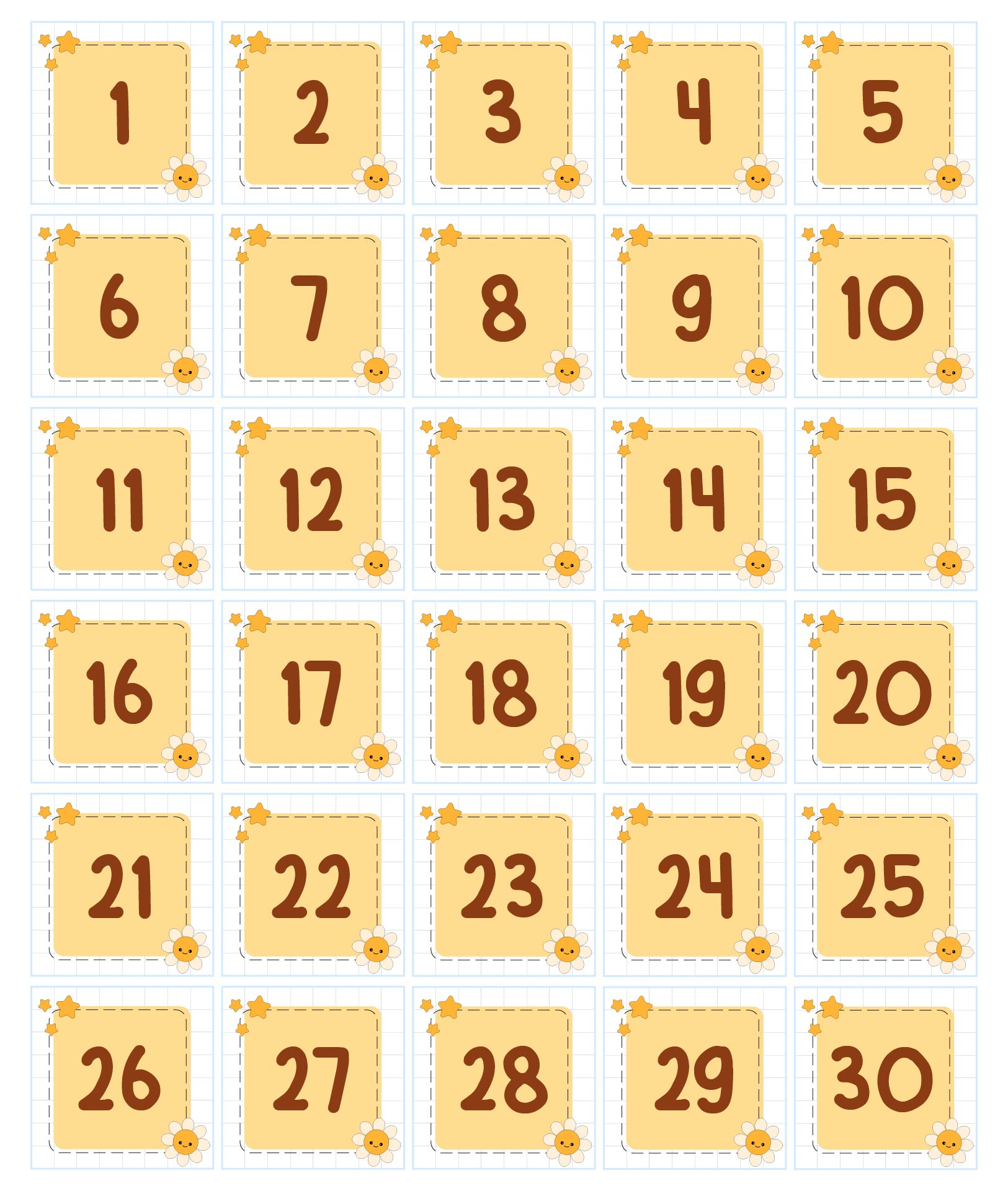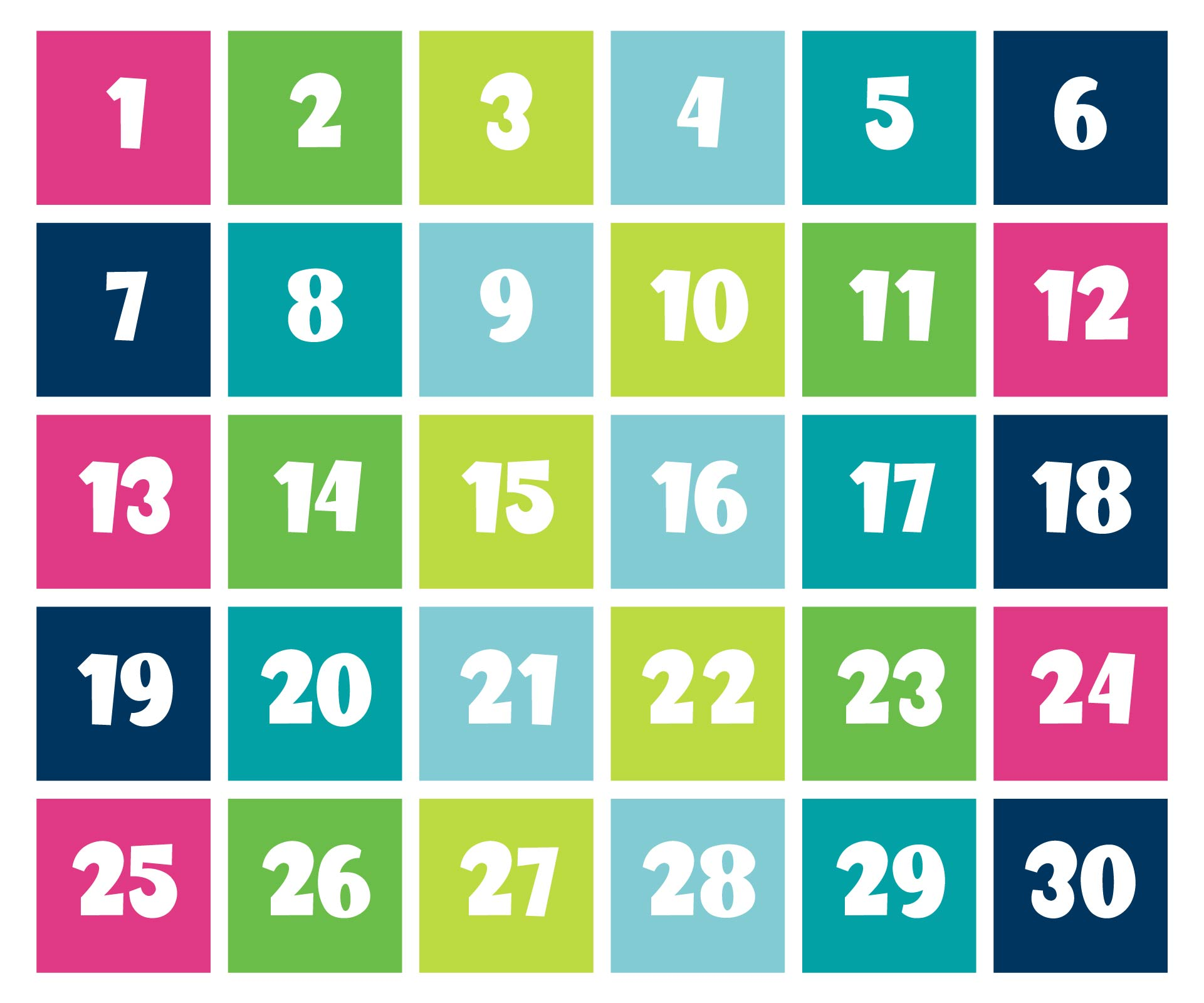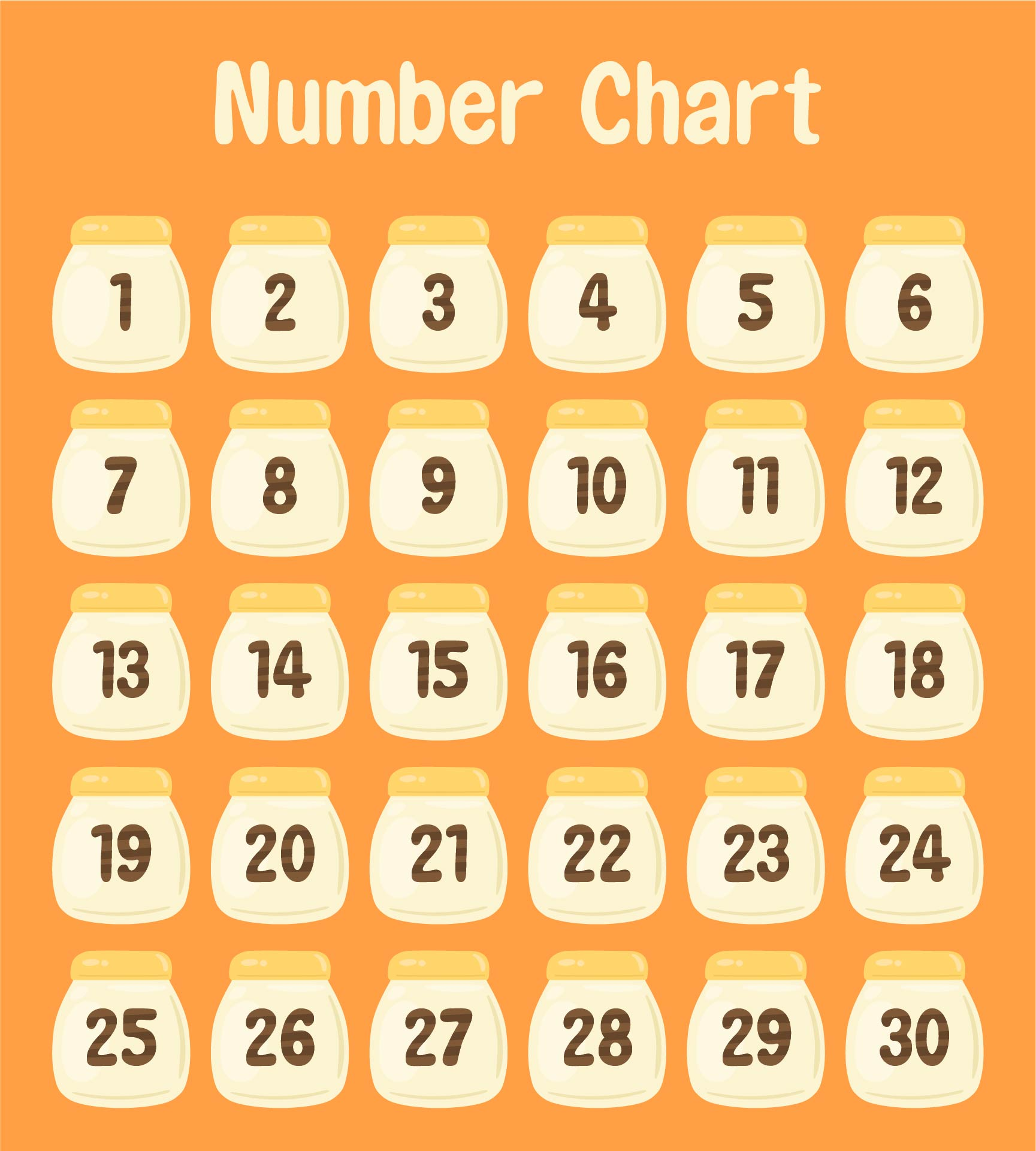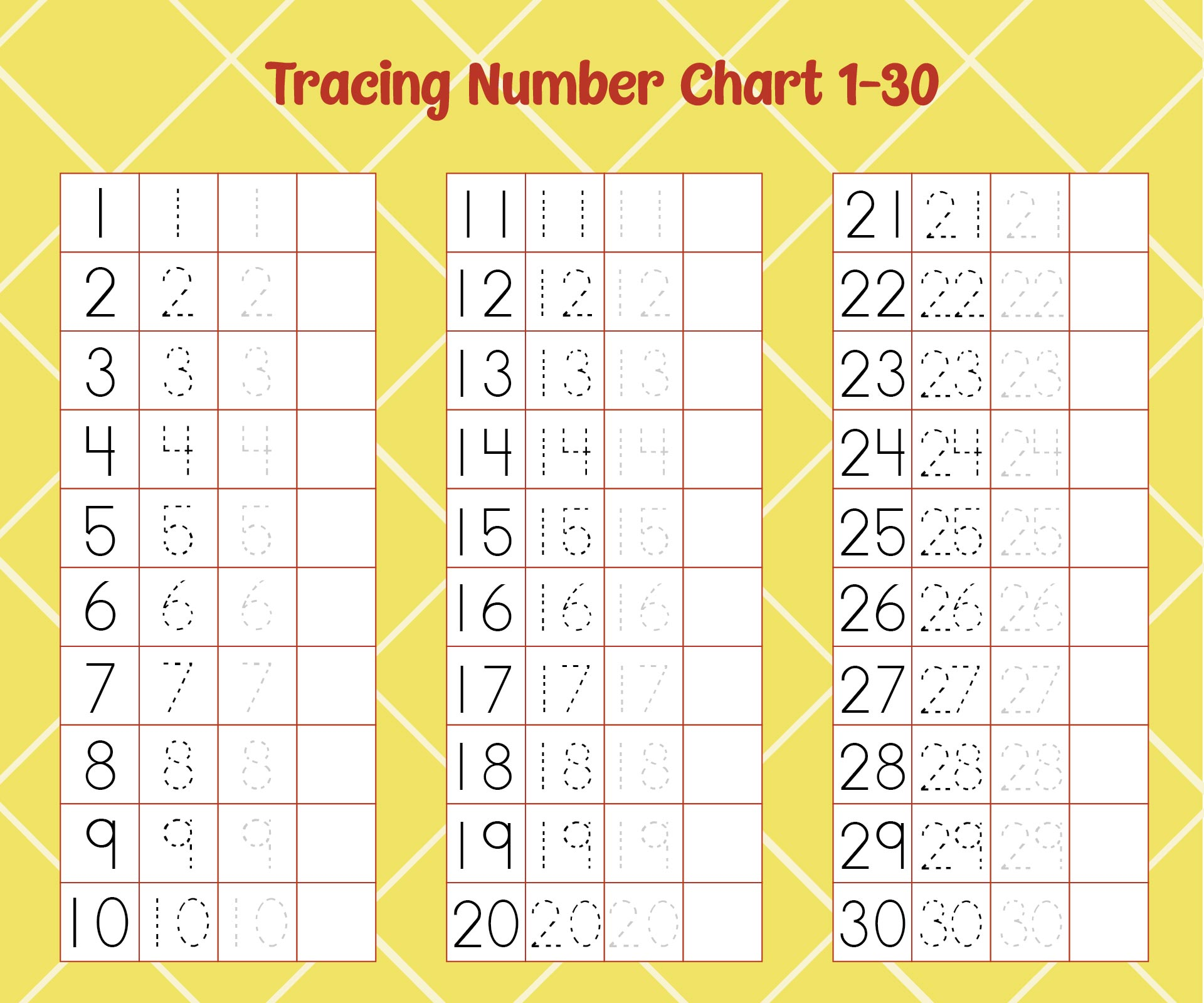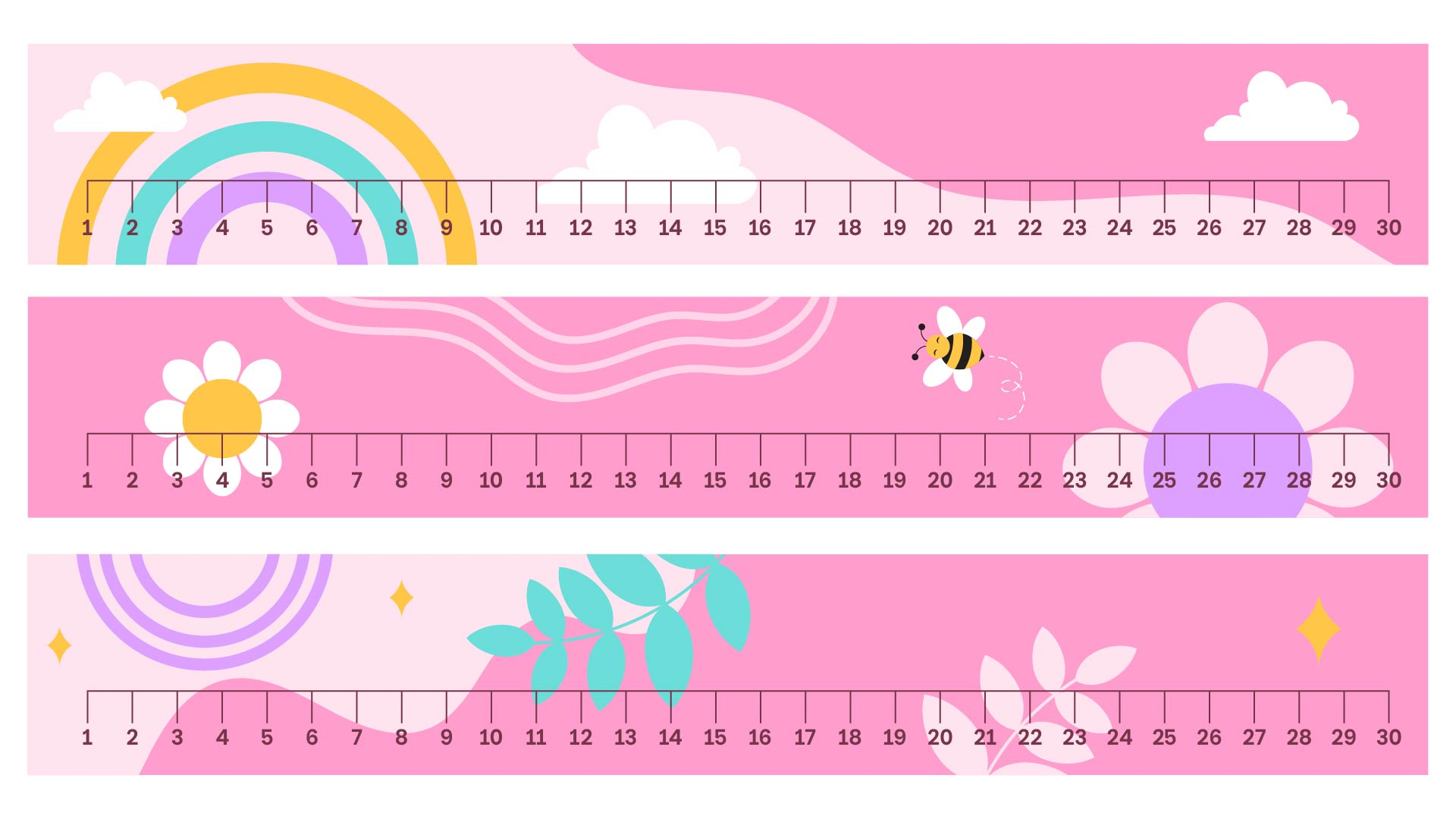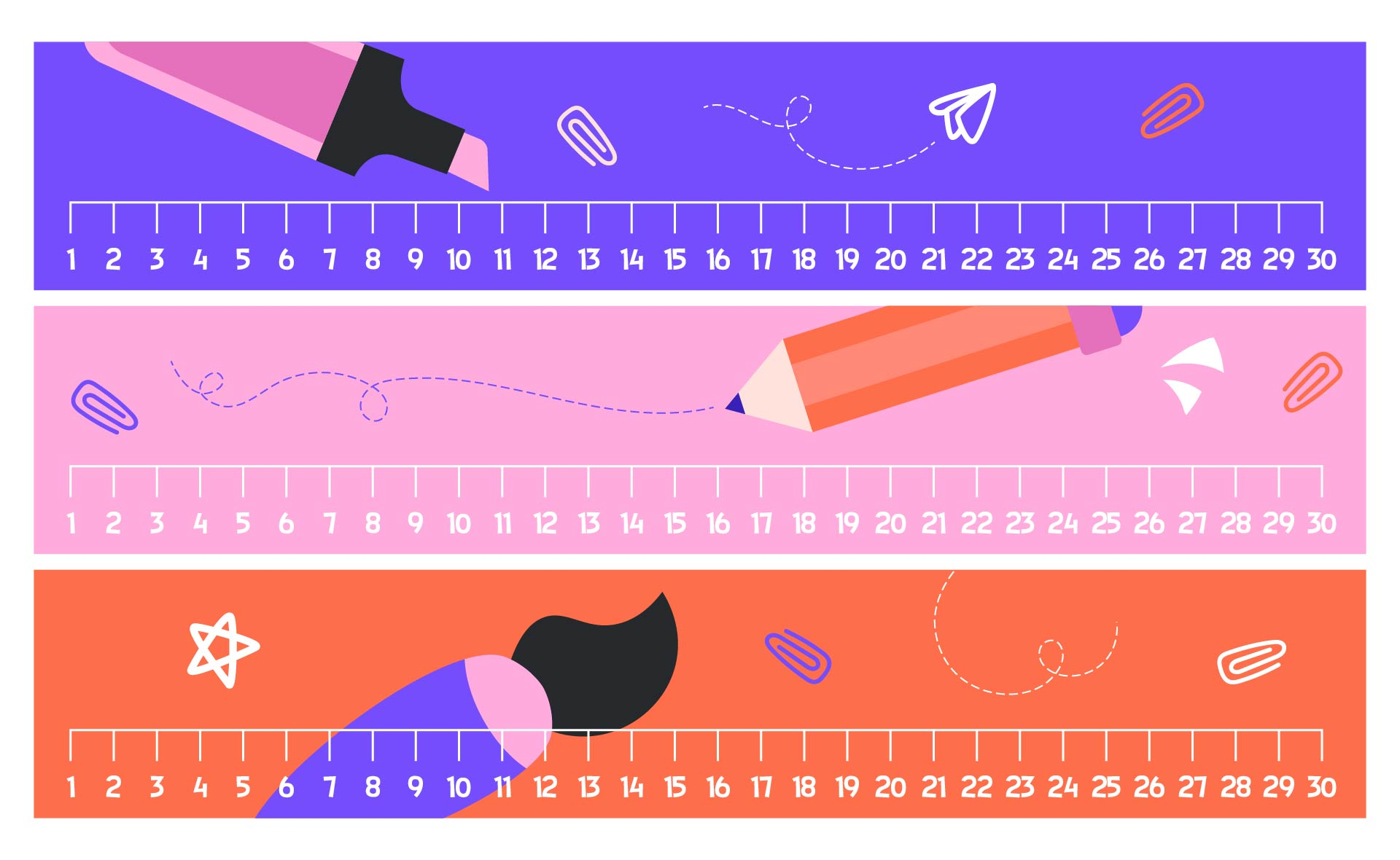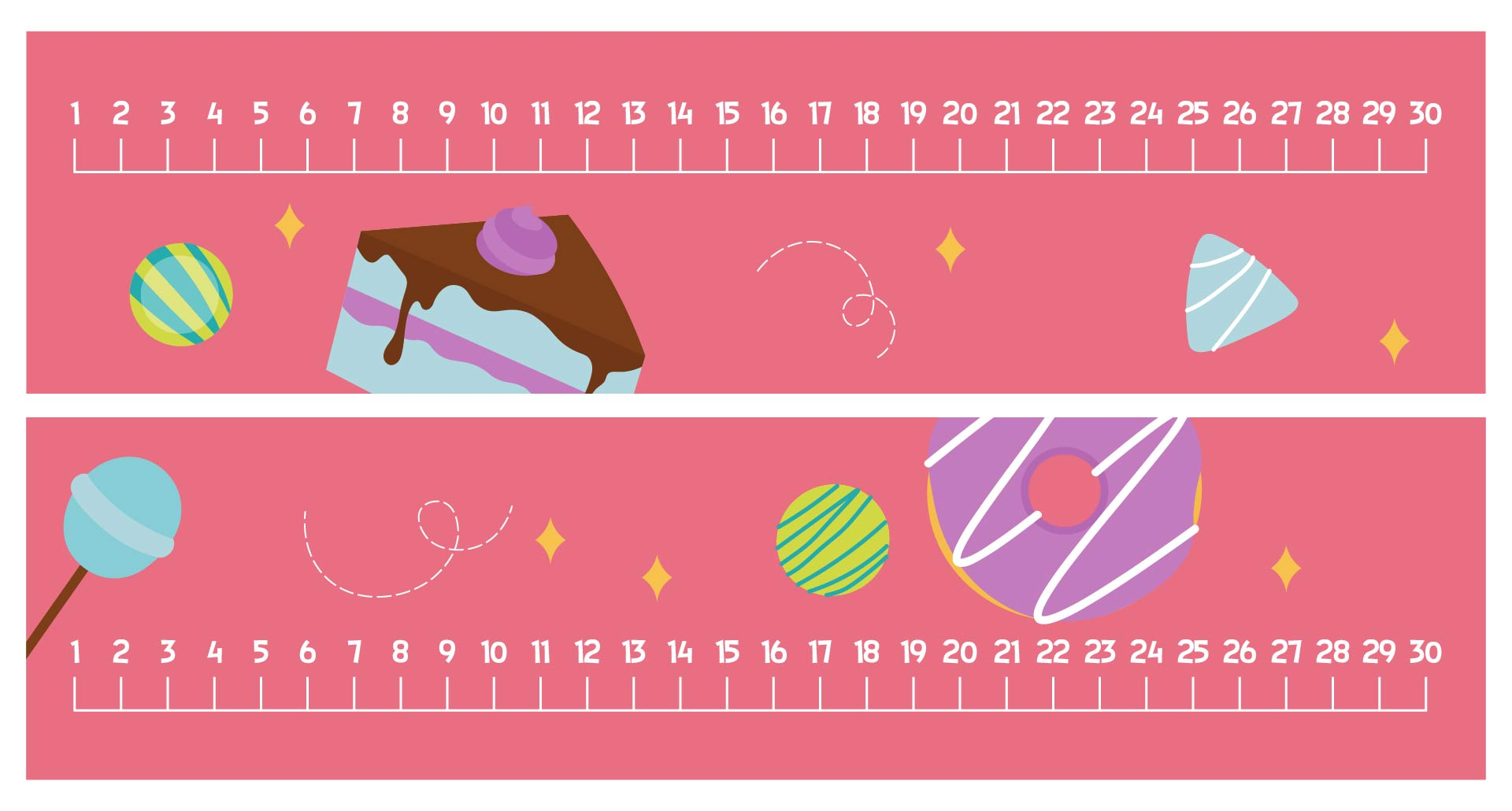## What are the Benefits of Basic Mathematical Operations in Life?

Teaching kids basic math operations is very important to do because they can get many benefits. Some of those benefits are as follows.

• Basic math operations like fraction and decimals can help children to develop their logical thinking. With good logical skills, children will be able to solve various problems in everyday life. They will also be able to make the right decisions from every day-to-day problem.

• Children can also build a systematic mindset if they master these basic mathematical operations. This systematic mindset is needed to do schoolwork and daily tasks.

Meanwhile, basic mathematical operations are also useful for your everyday life. Some of them are as follows.

• Basic math operations can make you a patient person. That's because questions of basic math operations require high focus and patience to be answered correctly. By calculating mathematical operations regularly, your patience will also be trained. You can also improve your concentration ability.

• Basic math operations can give you good profit and loss knowledge. An example is when you sell or buy items. These buying and selling transactions require knowledge of basic mathematical operations. Buying and selling transactions are often carried out in everyday life, so you must master the knowledge of basic mathematical operations.

• Basic mathematical operations are also able to improve critical and creative thinking. The reason is, mathematics teaches humans to think logically and critically. It will make humans able to think correctly every day. Therefore, people who have mastered basic mathematical operations will be good at arithmetic and able to analyze problems objectively.

• Basic mathematical operations are also the foundation of various other sciences. An example is the health sciences. Basic mathematical operations can help health workers to calculate the body mass index of patients. This is useful for determining normal body weight and its relationship to the patient's health condition.